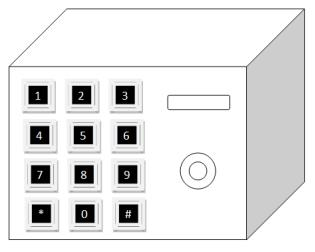favorite We need a little bit of your help to keep things running, click on this banner to learn more
Competitions

# Unlock my safeI do not remember how long my password is. Hence, you need to try a different length of the password. However, there are some hints that I can recall.

• I never use characters *, #, 0 and 9 in my password.
• Each digit in the password is distinct. That is, they never appear more than once.
• My password is at most 8 digits (1n8, where n is a number of digits in the password).
• Each digit i in the password always has the value less than or equal to n (that is, a password 132 is valid for n = 3 but a password such as 124 is invalid because the third digit exceeds 3).

Use the information above and generate all possible permutations. One permutation corresponds to one guess of a password to unlock my safe. Importantly, the correct password is deliberately fixed at position L \ 3 in the sorted array of permutations, where L is a number of all possible permutations and \ is an integer division. The sorted array of permutations is in ascending order and the starting index in the sorted array begins at 0 (not 1).

Write a program to find a correct password for a given length (a number of digits in the password).

#### Input

The first line contains an integer t (1t6) denoting the number of test cases. After that t test cases follow. Each test case contains an integer n (1n8) denoting a number of digits in a password.

### Explanation

There are 3 test cases above. In the second case, for example, the sorted permutations are {123, 132, 213, 231, 312, 321}. Password is located at the position 6 \ 3 = 2 (integer division). When the starting index begins at 0, the password is, therefore, 213.

Time limit 1 seconds
Memory limit 128 MiB
Input example #1
3
2
3
1

Output example #1
12
213
1

Author Dr. Apichat Heednakram
Source 2013 ACM-ICPC Thailand Southern Programming Contest, August 10, Problem A